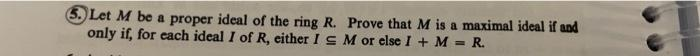# (Solved): 5. Let \( M \) be a proper ideal of the ring \( R \). Prove that \( M \) is a maximal ideal if and ...5. Let \( M \) be a proper ideal of the ring \( R \). Prove that \( M \) is a maximal ideal if and only if, for each ideal \( I \) of \( R \), either \( I \subseteq M \) or else \( I+M=R \).

We have an Answer from Expert